Courses

# Retro (Past 13 Years) JEE Main (Equilibrium)

## 16 Questions MCQ Test Chemistry for JEE Advanced | Retro (Past 13 Years) JEE Main (Equilibrium)

Description
This mock test of Retro (Past 13 Years) JEE Main (Equilibrium) for JEE helps you for every JEE entrance exam. This contains 16 Multiple Choice Questions for JEE Retro (Past 13 Years) JEE Main (Equilibrium) (mcq) to study with solutions a complete question bank. The solved questions answers in this Retro (Past 13 Years) JEE Main (Equilibrium) quiz give you a good mix of easy questions and tough questions. JEE students definitely take this Retro (Past 13 Years) JEE Main (Equilibrium) exercise for a better result in the exam. You can find other Retro (Past 13 Years) JEE Main (Equilibrium) extra questions, long questions & short questions for JEE on EduRev as well by searching above.
QUESTION: 1

Solution:
QUESTION: 2

Solution:
QUESTION: 3

### A vessel at 1000 K contains CO2 with a pressure of 0.5 atm. Some of the CO2 is converted into CO on the addition of graphite. If the total pressure at equilibrium is 0.8 atm, the value of Kp is  (AIEEE 2011)

Solution:
QUESTION: 4

For the following three reactions I, II and III, equilibrium constants are given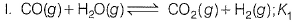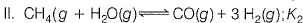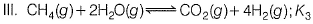Q. Which of the following relations is correct?

(AIEEE 2008)

Solution:
QUESTION: 5

The equilibrium constants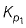and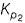for the reactions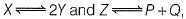, respectively are in the ratio of 1 : 9. If the degree of dissociation of X and Z be equal, then the ratio of total pressure at these equilibria is

(AIEEE 2008)

Solution:
QUESTION: 6

The Equilibrium constant Kc for the reaction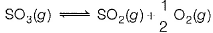is 4.9x 10-2. The value of Kc for the reaction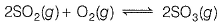Will be

(AIEEE 2006)

Solution:
QUESTION: 7

Phosphorus pentachloride dissociates as follows, in a closed reaction vessel,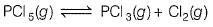If total pressure, at equilibrium, of the reaction mixture is p and degree of dissociation of PCI5 is x, the partial pressure Of PCI3 Will be

(AIEEE 2006)

Solution:
QUESTION: 8

An amount of solid NH4HS is placed in a flask already containing ammonia gas at a certain temperature and 0.50 atm pressure. Ammonium hydrogen sulphide decomposes to yield NH3 and H2S gases in the flask.
When the decomposition reaction reaches equilibrium, the total pressure in the flask rises to 0.84 atm. The equilibrium constant for the decomposition of NH4HS at this temperature is

(AIEEE 2005)

Solution:
QUESTION: 9

For the reaction,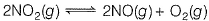[Kc = 1.8x 10-6 at 184°C, R = 0.00831 kJ/(mol K)]

When Kp and Kc are compared at 184°C it is found that

(AIEEE 2005)

Solution:
QUESTION: 10

The exothermic formation of CIF3 is represented by the equation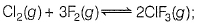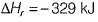Q. Which of the following will increase the quantity of CIF3 in an equilibrium mixture of Cl2, F2 and CIF3?

Solution:
QUESTION: 11

What is the equilibrium expression for the reaction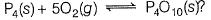(A1EEE 2004)

Solution:
QUESTION: 12

For the reaction,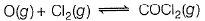the Kp / Kc is equal to

(AIEEE 2004)

Solution:
QUESTION: 13

For the reaction equilibrium,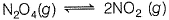the concentrations of N2O4 and NO2 at equilibrium are 4.8 x 10-2 and 1.2 x 10-2 mol L-1, respectively. The value of Kc for the reaction is

(AIEEE 2003)

Solution:
QUESTION: 14

Consider the reaction equilibrium,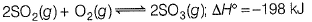On the basis of Le-Chatelier’s principle, the condition favourable for the forward reaction is

(AIEEE 2003)

Solution:
QUESTION: 15

For the following reaction in gaseous phase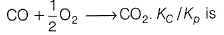(AIEEE 2002)

Solution:
QUESTION: 16

One of the following equilibria is not affected by change in volume of the flask

(AIEEE 2002)

Solution: